## Consider the motion of a 4.00-kg particle that moves with potential energy given by U(x) = + a) Suppose the particle is moving with a speed

Question

Consider the motion of a 4.00-kg particle that moves with potential energy given by U(x) = + a) Suppose the particle is moving with a speed of 3.00 m/s when it is located at x = 1.00 m. What is the speed of the object when it is located at x = 5.00 m? b) What is the magnitude of the force on the 4.00-kg particle when it is located at x = 5.00 m?

in progress 0
6 months 2021-07-29T23:51:22+00:00 1 Answers 57 views 0

## Answers ( )

1. Correct question:

Consider the motion of a 4.00-kg particle that moves with potential energy given by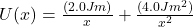a) Suppose the particle is moving with a speed of 3.00 m/s when it is located at x = 1.00 m. What is the speed of the object when it is located at x = 5.00 m?

b) What is the magnitude of the force on the 4.00-kg particle when it is located at x = 5.00 m?

a) 3.33 m/s

b) 0.016 N

Explanation:

a) given:

V = 3.00 m/s

x1 = 1.00 m

x = 5.00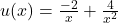At x = 1.00 m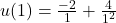= 4J

Kinetic energy = (1/2)mv²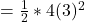= 18J

Total energy will be =

4J + 18J = 22J

At x = 5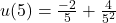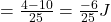= -0.24J

Kinetic energy =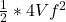= 2Vf²

Total energy =

2Vf² – 0.024

Using conservation of energy,

Initial total energy = final total energy

22 = 2Vf² – 0.24

Vf² = (22+0.24) / 2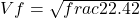= 3.33 m/s

b) magnitude of force when x = 5.0m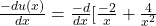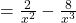At x = 5.0 m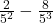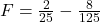= 0.016N